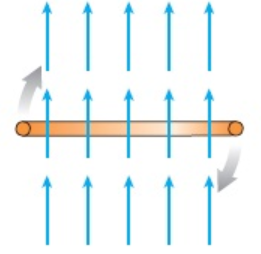# Problem: (Figure 1) shows a 100-turn coil of wire of radius 11cm in a 0.35T magnetic field. The coil is rotated 90° in 0.30 s, ending up parallel to the field. What is the average emf induced in the coil as it rotates?

###### FREE Expert Solution

The average emf induced into the coil is given by:

$\overline{){\mathbf{\epsilon }}{\mathbf{=}}{\mathbf{N}}\frac{\mathbf{∆}\mathbf{\varphi }}{\mathbf{∆}\mathbf{t}}}$, where Φ  is the magnetic flux, N is the number of turns, and t is the time.

Magnetic flux:

$\overline{){{\mathbf{\varphi }}}_{{\mathbf{B}}}{\mathbf{=}}{\mathbf{B}}{\mathbf{A}}{\mathbf{c}}{\mathbf{o}}{\mathbf{s}}{\mathbf{\theta }}}$, where B is the magnetic field, A is the cross-sectional area of the coil, and θ is the angle between the plane of the coil and the magnetic field.

98% (1 ratings)###### Problem Details

(Figure 1) shows a 100-turn coil of wire of radius 11cm in a 0.35T magnetic field.The coil is rotated 90° in 0.30 s, ending up parallel to the field. What is the average emf induced in the coil as it rotates?